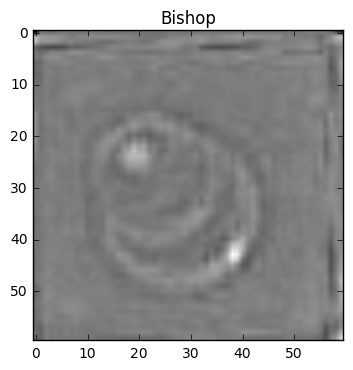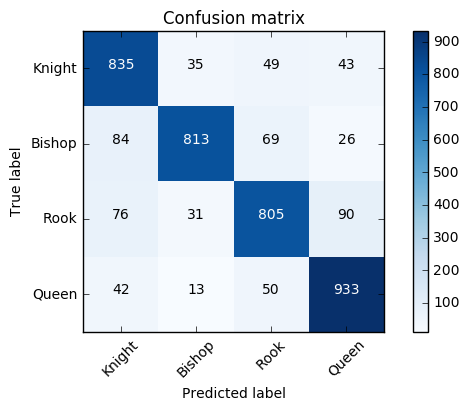# Classification of Promotable Chess Pieces¶In this notebook, the goal is to use TensorFlow to train a convolutional neural network to classify images as either being a knight, bishop, rook, or queen. The goal is to use the model to determine which piece a player promoted their pawn to when playing against the Raspberry Turk. In most cases players will promote to a queen, so the robot could assume that, but there are many examples when underpromotion is a better option.

In :
```import project
from raspberryturk.core.data.dataset import Dataset
import numpy as np
import tensorflow as tf
from random import randint
```
In :
```MAX_DISPLAY_STEP = 1000
LEARNING_RATE = 1e-4
TRAINING_ITERATIONS = 10000
DROPOUT = 0.5
START_BATCH_SIZE = 5
END_BATCH_SIZE = 100
```

## Data¶

This dataset, `dataset7.npz`, was created using `create_dataset.py`. It includes 19,968 ZCA whitened grayscale images of only promotable pieces.

In :
```d = Dataset.load_file(project.path('data', 'processed', 'dataset7.npz'))
```
In :
```labels_count = d.y_train.shape
image_size = 3600
image_width = 60
image_height = 60
labels = ['Knight', 'Bishop', 'Rook', 'Queen']
```
In :
```train_images = d.X_train
train_labels = d.y_train
validation_images = d.X_val
validation_labels = d.y_val
```
In :
```import matplotlib.pyplot as plt
from matplotlib import cm
piece_index = 0
img = train_images[piece_index].reshape(image_width, image_height)
plt.title(labels[train_labels[piece_index].argmax()])
plt.imshow(img, cmap=cm.gray)
plt.show()
```## Model¶

The model consists of 2 convolutional layers, each with using ReLU activation function and a max pool layer after each one. This is followed by a fully connected layer with 1024 hidden units, and finally a softmax layer. Dropout is used for regularization, and loss is defined as the cross entropy between the prediction label vector and the correct label vector.

In :
```def weight_variable(shape):
initial = tf.truncated_normal(shape, stddev=0.1)
return tf.Variable(initial)

def bias_variable(shape):
initial = tf.constant(0.1, shape=shape)
return tf.Variable(initial)

def conv2d(x, W):

def max_pool_2x2(x):
```
In :
```x = tf.placeholder('float', shape=[None, image_size])
y_ = tf.placeholder('float', shape=[None, labels_count])

W_conv1 = weight_variable([5, 5, 1, 32])
b_conv1 = bias_variable()

image = tf.reshape(x, [-1, image_width, image_height, 1])

h_conv1 = tf.nn.relu(conv2d(image, W_conv1) + b_conv1)
h_pool1 = max_pool_2x2(h_conv1)

W_conv2 = weight_variable([5, 5, 32, 64])
b_conv2 = bias_variable()

h_conv2 = tf.nn.relu(conv2d(h_pool1, W_conv2) + b_conv2)
h_pool2 = max_pool_2x2(h_conv2)

W_fc1 = weight_variable([15 * 15 * 64, 1024])
b_fc1 = bias_variable()

h_pool2_flat = tf.reshape(h_pool2, [-1, 15 * 15 * 64])
h_fc1 = tf.nn.relu(tf.matmul(h_pool2_flat, W_fc1) + b_fc1)

keep_prob = tf.placeholder('float')
h_fc1_drop = tf.nn.dropout(h_fc1, keep_prob)

W_fc2 = weight_variable([1024, labels_count])
b_fc2 = bias_variable([labels_count])

y = tf.nn.softmax(tf.matmul(h_fc1_drop, W_fc2) + b_fc2)

cross_entropy = -tf.reduce_sum(y_*tf.log(y))

correct_prediction = tf.equal(tf.argmax(y,1), tf.argmax(y_,1))
accuracy = tf.reduce_mean(tf.cast(correct_prediction, 'float'))

predict = tf.argmax(y, 1)
```

## Batching¶

Use stocastic gradient descent for faster training.

In :
```epochs_completed = 0
index_in_epoch = 0
num_examples = d.X_train.shape

def next_batch(batch_size):
global train_images
global train_labels
global index_in_epoch
global epochs_completed

start = index_in_epoch
index_in_epoch += batch_size

if index_in_epoch > num_examples:
epochs_completed += 1
perm = np.arange(num_examples)
np.random.shuffle(perm)
train_images = train_images[perm]
train_labels = train_labels[perm]
start = 0
index_in_epoch = batch_size
assert batch_size <= num_examples
end = index_in_epoch
return train_images[start:end], train_labels[start:end]
```

## Training¶

Begin training, output the training/validation error at each display step.

In :
```init = tf.global_variables_initializer()
sess = tf.InteractiveSession()

sess.run(init)
saver = tf.train.Saver()
```
In :
```train_accuracies = []
validation_accuracies = []
x_range = []

display_step = 10

previous_best = 0.0
for i in range(TRAINING_ITERATIONS):
batch_size = int((END_BATCH_SIZE - START_BATCH_SIZE) * float(i) / TRAINING_ITERATIONS) + 5
batch_xs, batch_ys = next_batch(batch_size)
if i%display_step == 0 or (i+1) == TRAINING_ITERATIONS:
train_accuracy = accuracy.eval(feed_dict={x: batch_xs,
y_: batch_ys,
keep_prob: 1.0})
val_len = 1000
ind = randint(0, validation_images.shape - val_len - 1)
val_images_batch = validation_images[ind:ind+val_len]
val_labels_batch = validation_labels[ind:ind+val_len]
validation_accuracy = accuracy.eval(feed_dict={x: val_images_batch,
y_: val_labels_batch,
keep_prob: 1.0})

print('training_accuracy / validation_accuracy => %.2f / %.2f for step %d'%(train_accuracy, validation_accuracy, i))
train_accuracies.append(train_accuracy)
validation_accuracies.append(validation_accuracy)
x_range.append(i)
if validation_accuracy > previous_best:
previous_best = validation_accuracy
if i%(display_step*10) == 0 and i:
display_step = min(MAX_DISPLAY_STEP, display_step * 10)

sess.run(train_step, feed_dict={x: batch_xs, y_: batch_ys, keep_prob: DROPOUT})
print("Finished training, best batch validation model accuracy: %.5f"%(previous_best))
```
```training_accuracy / validation_accuracy => 0.60 / 0.24 for step 0
training_accuracy / validation_accuracy => 0.20 / 0.23 for step 10
training_accuracy / validation_accuracy => 0.40 / 0.26 for step 20
training_accuracy / validation_accuracy => 0.40 / 0.28 for step 30
training_accuracy / validation_accuracy => 0.00 / 0.29 for step 40
training_accuracy / validation_accuracy => 0.40 / 0.24 for step 50
training_accuracy / validation_accuracy => 0.00 / 0.28 for step 60
training_accuracy / validation_accuracy => 0.20 / 0.26 for step 70
training_accuracy / validation_accuracy => 0.40 / 0.29 for step 80
training_accuracy / validation_accuracy => 0.00 / 0.27 for step 90
training_accuracy / validation_accuracy => 0.40 / 0.28 for step 100
training_accuracy / validation_accuracy => 0.17 / 0.31 for step 200
training_accuracy / validation_accuracy => 0.43 / 0.30 for step 300
training_accuracy / validation_accuracy => 0.38 / 0.30 for step 400
training_accuracy / validation_accuracy => 0.22 / 0.31 for step 500
training_accuracy / validation_accuracy => 0.50 / 0.34 for step 600
training_accuracy / validation_accuracy => 0.18 / 0.36 for step 700
training_accuracy / validation_accuracy => 0.33 / 0.34 for step 800
training_accuracy / validation_accuracy => 0.38 / 0.36 for step 900
training_accuracy / validation_accuracy => 0.36 / 0.34 for step 1000
training_accuracy / validation_accuracy => 0.62 / 0.50 for step 2000
training_accuracy / validation_accuracy => 0.82 / 0.59 for step 3000
training_accuracy / validation_accuracy => 0.95 / 0.69 for step 4000
training_accuracy / validation_accuracy => 1.00 / 0.76 for step 5000
training_accuracy / validation_accuracy => 0.98 / 0.81 for step 6000
training_accuracy / validation_accuracy => 1.00 / 0.83 for step 7000
training_accuracy / validation_accuracy => 1.00 / 0.82 for step 8000
training_accuracy / validation_accuracy => 1.00 / 0.84 for step 9000
training_accuracy / validation_accuracy => 1.00 / 0.85 for step 9999
Finished training, best batch validation model accuracy: 0.85300
```

## Validation¶

Determine validation accuracy for the trained model, and produce a confusion matrix to visualize the effectiveness of training for the task of classification.

In :
```pred = sess.run(predict, feed_dict={x: validation_images, keep_prob: 1.0})

full_validation_accuracy = accuracy.eval(feed_dict={x: validation_images,
y_: validation_labels,
keep_prob: 1.0})
print "Validation accuracy: %.5f" % (full_validation_accuracy)
```
```Validation accuracy: 0.84777
```
In :
```from sklearn.metrics import confusion_matrix

conf = confusion_matrix(validation_labels.argmax(axis=1), pred)
```
In :
```from helpers import plot_confusion_matrix

plot_confusion_matrix(conf, labels)
```In :
```sess.close()
```

The full source of this notebook can be found on GitHub.